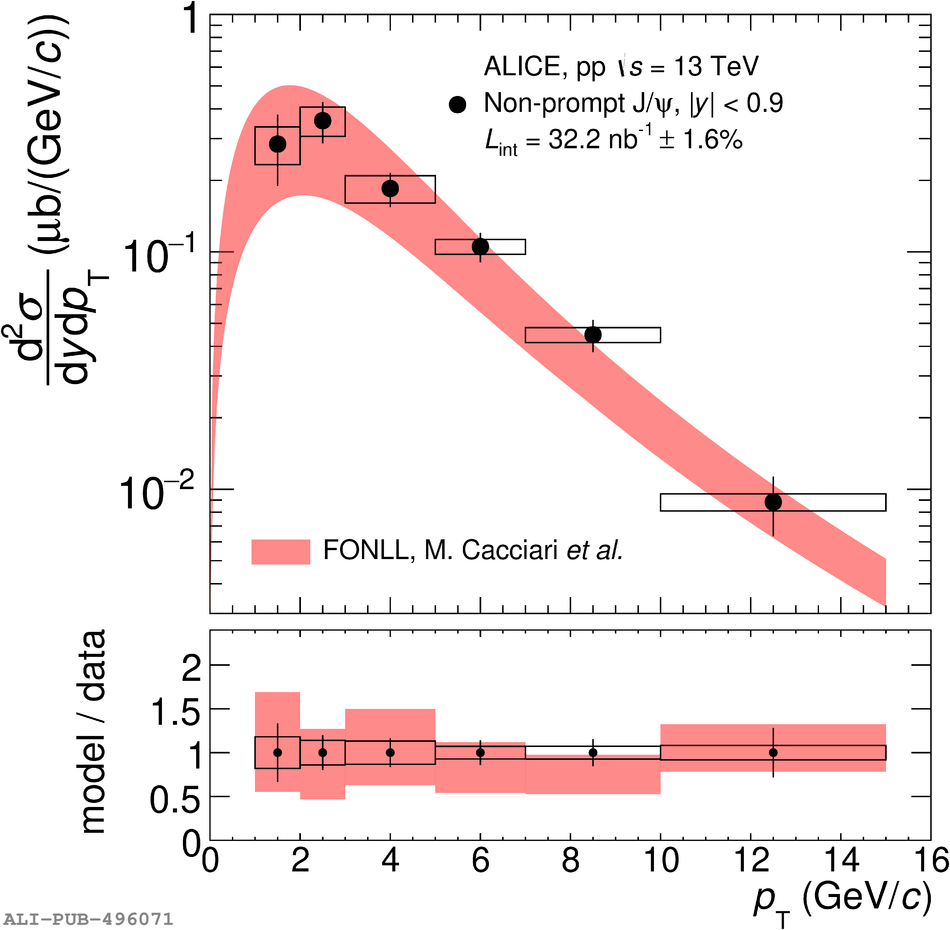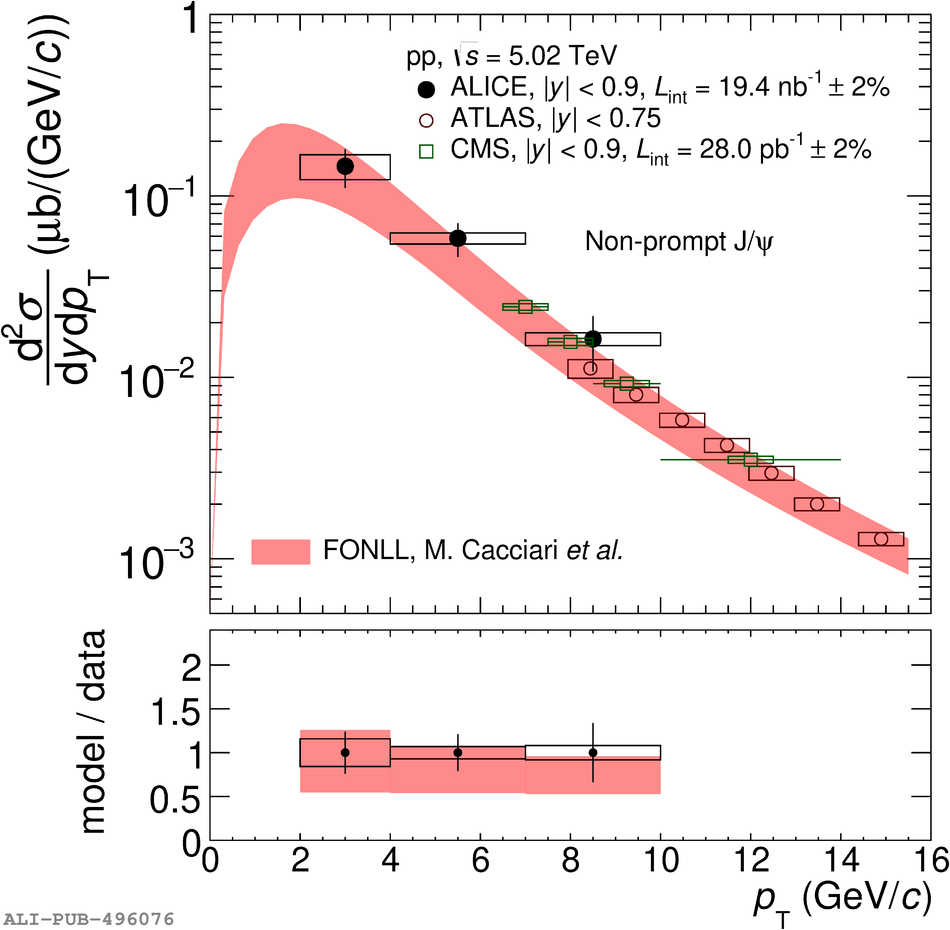# Figure 3

 The $\frac{{\rm d}^2\sigma}{{\rm d}y {\rm d}\pt}$ of non-prompt \pJPsi\ in pp collisions at $\sqrt{s}$ = 13 TeV (left panel) and 5.02 TeV (right panel) as a function of \pt. The measurement at \s = 5.02 TeV is compared with similar measurements from CMS  and ATLAS  collaborations at high \pt The error bars (boxes) represent the statistical (systematic) uncertainties. Uncertainties due to the luminosity are not included in the boxes, except in the case of ATLAS. The results are compared with the FONLL calculations  at both energies. Bottom panels show the ratios FONLL to ALICE data. The uncertainty band represents the relative uncertainty from the model whereas the points centered around unity refer to relative statistical and systematic uncertainties on ALICE data points.